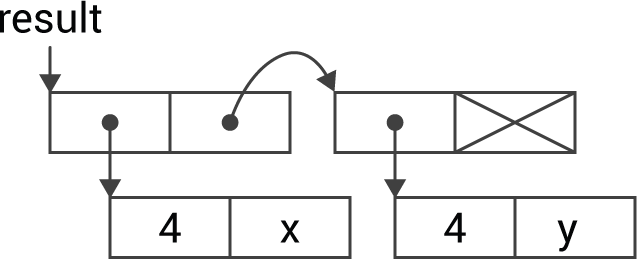## Converting between C and uLisp

This article summarises how to convert values between C variables of different types and the corresponding uLisp objects.

#### Update

27th March 2023: The examples have been updated to uLisp Release 4.4.

### Integers

#### C to uLisp

The built-in function number() takes an int argument and returns a uLisp integer object.

For example, here's the definition of the uLisp function (millis) which returns the value of the Arduino function millis():

```object *fn_millis (object *args, object *env) {
(void) args, (void) env;
return number(millis());
}```

#### uLisp to C

The built-in function checkinteger() takes a uLisp object and returns a C int. It causes an error if the parameter isn't a uLisp integer.

For example, here's the definition of the uLisp function (delay n) which takes one integer parameter and calls the Arduino function delay(n). It returns n:

```object *fn_delay (object *args, object *env) {
(void) env;
object *arg1 = first(args);
delay(checkinteger(arg1));
return arg1;
}```

### Floats

#### C to uLisp

On 32-bit platforms the built-in function makefloat() takes a float argument and returns a uLisp floating-point object.

For example the following function implements the uLisp function sin:

```object *fn_sin (object *args, object *env) {
(void) env;
return makefloat(sin(checkintfloat(first(args))));
}```

#### uLisp to C

On 32-bit platforms the built-in function checkintfloat() takes a uLisp object and returns a C int or a C float. It causes an error if the parameter isn't a uLisp integer or floating-point number.

For example, you could define a uLisp function radians that converted a Lisp number from degrees to radians as follows:

```object *fn_radians (object *args, object *env) {
(void) env;
object* arg = first(args);
return makefloat(checkintfloat(arg) * 3.14159 / 180.0);
}```

### Characters

#### C to uLisp

The built-in function character() takes a char argument and returns a uLisp character object.

For example, here's the definition of the Lisp function code-char which takes a uLisp integer object and converts it to a uLisp character object:

```object *fn_codechar (object *args, object *env) {
(void) env;
return character(checkinteger(first(args)));
}```

#### uLisp to C

The built-in function checkchar() takes a uLisp object and returns a C integer. It causes an error if the parameter isn't a uLisp character object.

For example, here's the definition of the Lisp function char-code which takes a uLisp character object and converts it to a uLisp integer object:

```object *fn_charcode (object *args, object *env) {
(void) env;
return number(checkchar(first(args)));
}```

### Strings

#### C to uLisp

The function lispstring() will take a C char* string and return a uLisp string object:

```object *lispstring (char *s) {
object *obj = newstring();
object *tail = obj;
while(1) {
char ch = *s++;
if (ch == 0) break;
if (ch == '\\') ch = *s++;
buildstring(ch, &tail);
}
return obj;
}```

It uses the function newstring() to start constructing a uLisp string, and buildstring() to add characters to the end of the string being constructed.

For example, here's the definition of the uLisp Wi-Fi extension function (wifi-localip) which returns the local ip address as a uLisp string:

```object *fn_wifilocalip (object *args, object *env) {
(void) args, (void) env;
return lispstring((char*)WiFi.localIP().toString().c_str());
}```

#### uLisp to C

The function cstring() takes a uLisp string, a buffer, and the buffer length, and returns the buffer containing the uLisp string converted to a C string:

```char *cstring (object *form, char *buffer, int buflen) {
form = cdr(checkstring(form));
int index = 0;
while (form != NULL) {
int chars = form->integer;
for (int i=(sizeof(int)-1)*8; i>=0; i=i-8) {
char ch = chars>>i & 0xFF;
if (ch) {
if (index >= buflen-1) error2(PSTR("no room for string"));
buffer[index++] = ch;
}
}
form = car(form);
}
buffer[index] = '\0';
return buffer;
}```

It first calls checkstring() to check that the argument is a Lisp string.

### Lists

This section explains how to convert between a uLisp list and multiple C values such as in variables, a struct, or an array.

#### C to uLisp

As an example of converting multiple values between C and Lisp, here's an example of a C routine to read the x and y values from an analogue joystick connected to the analogue inputs A0 and A1. It uses pointers to pass out the values x and y from the joystick:

```void Joystick (int *x, int *y) {
}```

We will convert this to a uLisp function (joystick) which returns the x and y values as a list of two integers:

```> (joystick)
(512 250)```

I usually find it useful to draw a diagram of the Lisp structure I want to construct:The '4' label identifies an integer object. Here's the definition of the joystick function:

```object *fn_joystick (object *args, object *env) {
(void) args, (void) env;
int x, y;
Joystick(&x, &y);
object *result = cons(number(x), cons(number(y), NULL));
return result;
}```

#### uLisp to C

To transfer multiple values from uLisp to C one way is to write a function that takes a list argument. Here's a function servo that takes a list of two values and writes them to analogue outputs:

```object *fn_servo (object *args, object *env) {
(void) args, (void) env;
object *arg1 = first(args);
if (listlength(args) != 2) error(PSTR("argument is not a list of two items"));  pinMode(5, OUTPUT); pinMode(6, OUTPUT);
analogWrite(5, integer(first(arg1)));
analogWrite(6, integer(second(arg1)));
return arg1;
}```

The call to listlength() checks that the argument list contains two parameters. This isn't necessary if you are defining a function and have specified the number of parameters using the minmax code; see Adding your own functions - Minmax code.

To test the function you could write, for example:

```> (servo '(255 255))
(255 255)```